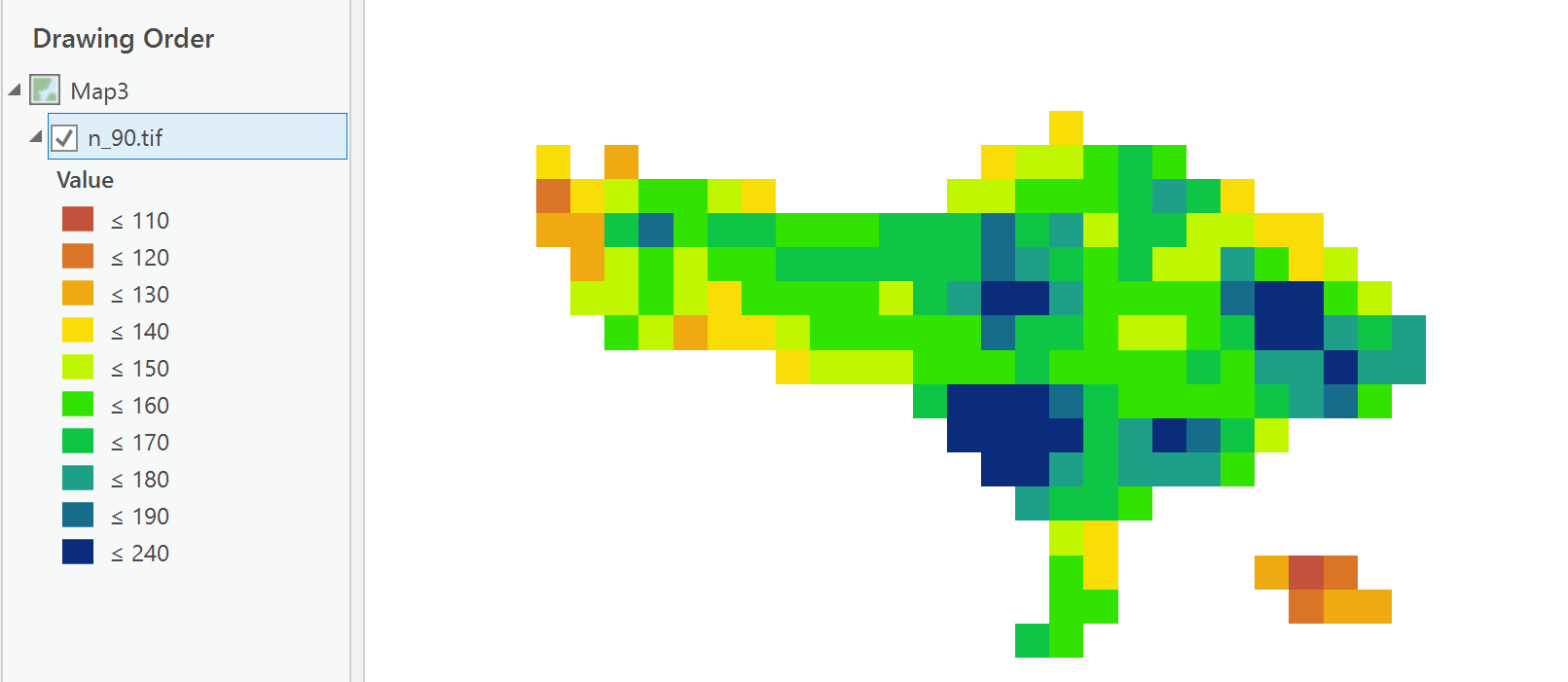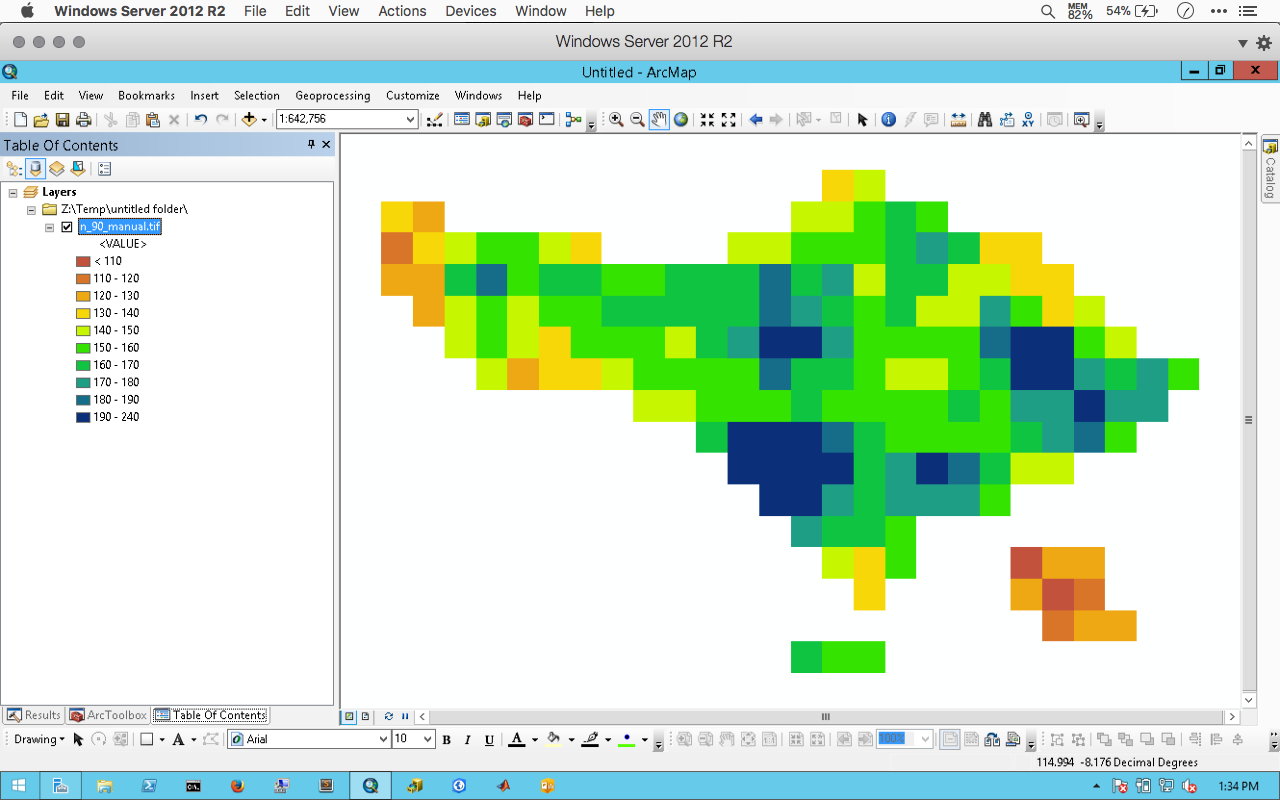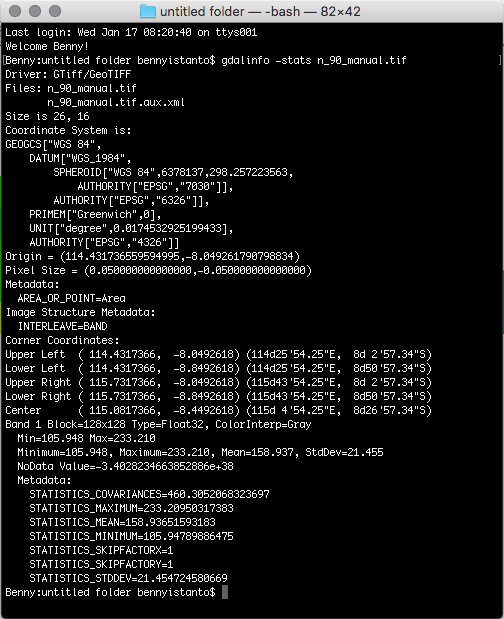# How to calculate the percentile for each cell from timeseries raster

3588
12
Jump to solution
01-16-2018 01:26 AMNew Contributor II

Branched from: https://community.esri.com/thread/95403

Hi Xander Bakker thank you for above script. I have tested and it works using my data.

But I have question. Let say I have timeseries raster data from 1981 to 2016, its the max of daily rainfall in a year, so its just 1 raster data for 1 year. For each cell in x,y location from 1981 to 2016, I would like to calculate the percentile of i.e 90%.

How to calculate the percentile for each cell from timeseries raster using your script?

1 Solution

Accepted SolutionsbyMVP Esteemed Contributor
``import numpyimport arcpyflder = r"C:\Temp\Max3days"   # where I unzipped your filesarcpy.env.workspace = flderrasters = arcpy.ListRasters()arrs = []for i in rasters:    arrs.append(arcpy.RasterToNumPyArray(i))a = np.array(arrs)# ---- create the masked arrays assuming 0.0 is the null/nodata value ----arrs_m = []for i in arrs:    m = np.where(a == 0, 1, 0)    am = np.ma.MaskedArray(i, mask=m)    arrs_m.append(am)a_m = np.array(arrs_m)n_90 = np.nanpercentile(a_m, 90., axis=0)""" ---- I would like the coordinates and cell size of the lower left corner !!! NumPyArrayToRaster (in_array,                     {lower_left_corner},                     {x_cell_size},                     {y_cell_size},                     {value_to_nodata})"""out = arcpy.NumPyArrayToRaster(n_90, value_to_nodata=0.0)out_name = "\\".join([flder, "n_90.tif"])out.save(out_name)‍‍‍‍‍‍‍‍‍‍‍‍‍‍‍‍‍‍‍‍‍‍‍‍‍‍‍‍‍‍‍‍‍‍‍‍‍‍‍‍‍‍‍‍‍‍‍‍‍‍‍‍‍‍‍‍‍‍‍‍‍‍‍‍‍``

Now if you could fill me in on what the values should look like, I can change stuff.

The attached is the nanpercentile at the 90% percentile for the array stack.

Keep me posted, I haven't had a look in Arc anything yet, so do not add it into a dataframe or map with other data, since it has no coordinate or cell size information yet,.

ADDENDUM

pretty coarse grid or a small area12 RepliesbyEsri Esteemed Contributor

I branched your question to another thread since it is not a zonal statistics operation but rather a pixel based analysis. As far as I know there is no tool that will determine the 90% percentile on a cell based analysis for a series of rasters. However, you could follow the example provided here: https://community.esri.com/thread/198969-trend-analysis-through-time-series-of-raster-data#comment-7...

This will loop through all the cells and read out the values of the different rasters. Then you can determine the 90% percentile in a separate function and write that to a new raster. If you have a set of sample data, I can help you tweak the script.byMVP Esteemed Contributor

Interesting, you just need to convert your rasters to numpy arrays (RasterToNumPyArray in arcpy)

``a = np.arange(9).reshape(3,3)  # ---- a sample simple array# ---- make some fake arrays representing different times -----a_s = []for i in range(10):    a_s.append(a + i)    a_n = np.array(a_s)  # --- stack them depth-wise ----frmt_(a_n, title='Ten arrays')  # ---- let's look at them in sequence ----Ten arrays...-shape (10, 3, 3), ndim 3  .  0  1  2    1  2  3    2  3  4    3  4  5    4  5  6    5  6  7    6  7  8    7  8  9    8  9 10   ....  .  3  4  5    4  5  6    5  6  7    6  7  8    7  8  9    8  9 10    9 10 11   10 11 12   11 12 13   ....  .  6  7  8    7  8  9    8  9 10    9 10 11   10 11 12   11 12 13   12 13 14   13 14 15   14 15 16   ....cell = a_n[:,0,0]  # ---- take the top left cell for all arrayscellarray([0, 1, 2, 3, 4, 5, 6, 7, 8, 9])np.percentile(cell, 50)  # ---- the median4.5np.percentile(cell, 25)  # ---- lower quartile2.25np.percentile(cell, 90)  # ---- 90th percentile8.0999999999999996‍‍‍‍‍‍‍‍‍‍‍‍‍‍‍‍‍‍‍‍‍‍‍‍‍‍‍‍‍``

Then you can do the percentile for all the cells at once.

``np.percentile(a_n, q=90., axis=0) array([[  8.1,   9.1,  10.1],       [ 11.1,  12.1,  13.1],       [ 14.1,  15.1,  16.1]])‍‍‍‍‍``

Read the docs associated with numpy percentile and scipy percentile... there are other controlling factors that can be put into play without the need to compile a complete ogive for each cell.New Contributor II

Thank you Dan Patterson and Xander Bakker for explanation. Unfortunately it's difficult for me to modified above python script with numpy.

So far I have try using gdal, I found a script from StackExchange "gdal_calc.py -A stack.vrt allBands=A --calc='nanpercentile(A.astype(int16),85,axis=0)' --outfile out.tif" and arcpy script mentioned in this discussion Pool of raster values to calculate percentile

I am getting an output using both script, however looks entirely wrong if I compared using manual calculation using Excel (I convert all raster data to csv, and calculate the percentile for each point using formula below)

The idea is to get the rainfall threshold and rainfall-triggered flood with return period, let say for 10 and 25 year. If my data is in excel format, I used =PERCENTILE(DATARANGE,0.9) for calculating the rainfall threshold for 10 year return period of rainfall-triggered flood.

I have upload example data of rainfall below.

Rainfall Timeseries 1981 - 2016byMVP Esteemed Contributor
``import numpyimport arcpyflder = r"C:\Temp\Max3days"   # where I unzipped your filesarcpy.env.workspace = flderrasters = arcpy.ListRasters()arrs = []for i in rasters:    arrs.append(arcpy.RasterToNumPyArray(i))a = np.array(arrs)# ---- create the masked arrays assuming 0.0 is the null/nodata value ----arrs_m = []for i in arrs:    m = np.where(a == 0, 1, 0)    am = np.ma.MaskedArray(i, mask=m)    arrs_m.append(am)a_m = np.array(arrs_m)n_90 = np.nanpercentile(a_m, 90., axis=0)""" ---- I would like the coordinates and cell size of the lower left corner !!! NumPyArrayToRaster (in_array,                     {lower_left_corner},                     {x_cell_size},                     {y_cell_size},                     {value_to_nodata})"""out = arcpy.NumPyArrayToRaster(n_90, value_to_nodata=0.0)out_name = "\\".join([flder, "n_90.tif"])out.save(out_name)‍‍‍‍‍‍‍‍‍‍‍‍‍‍‍‍‍‍‍‍‍‍‍‍‍‍‍‍‍‍‍‍‍‍‍‍‍‍‍‍‍‍‍‍‍‍‍‍‍‍‍‍‍‍‍‍‍‍‍‍‍‍‍‍‍``

Now if you could fill me in on what the values should look like, I can change stuff.

The attached is the nanpercentile at the 90% percentile for the array stack.

Keep me posted, I haven't had a look in Arc anything yet, so do not add it into a dataframe or map with other data, since it has no coordinate or cell size information yet,.

ADDENDUM

pretty coarse grid or a small areaNew Contributor II

Wow excellent!

The result almost same with manual version, slightly different on the minimum value (your script is 109.391 and manual version is 105.947, for the max is same. See pictures attached (map and statistics info from gdal).

The raster extent is not exactly same with yours, because its from excel - convert to point - convert to raster and clip with boundary. Here's the file: n_90_manual.zipAnd yes you are correct, its pretty coarse grid with 0.05 deg spatial resolution.

The data came from CHIRPS global precipitation data CHG - Data and this data is the best available global precipitation data with temporal resolution daily, 5days, 10days, monthly, 2monthly, 3monthly and annual from 1981 until now and free for public. So far this data are very useful for me when getting weather station data from govt is very difficult.

Thank you Dan PattersonbyMVP Esteemed Contributor

Glad it worked Benny, Other statistics can be rapidly derived from the data if needed.  Let me know if there is anything else that is required.New Contributor II

One more thing, the TIF output don't have any spatial reference. How to make it available automatically on the output during the calculation, so it's ready to overlay with other data.byMVP Esteemed Contributor

Lines 22-30... there is a commented out section that shows what is required to provide a spatial reference and cell size to the data.  On line 30, I didn't have all the information so I simply used specify a lower left corner using the 2nd coding example here http://pro.arcgis.com/en/pro-app/arcpy/functions/numpyarraytoraster-function.htm

arcpy.Point([long, lat])

and specify the cell size... both equal for square cells (ie x degrees, y degrees)New Contributor II

Hi Dan,

Pardon of my ignorance. After reading carefully, I can run the script.

For line 22-30, I added

``arcpy.NumPyArrayToRaster(n_90, arcpy.Point(114.4317366,-8.8492618), 0.049242234706753, 0.049242234706753, 0)out = arcpy.NumPyArrayToRaster(n_90, value_to_nodata=0)‍‍‍‍‍‍``

But the geotiff file still have not spatial reference. Which part I missed it?

Thank you.